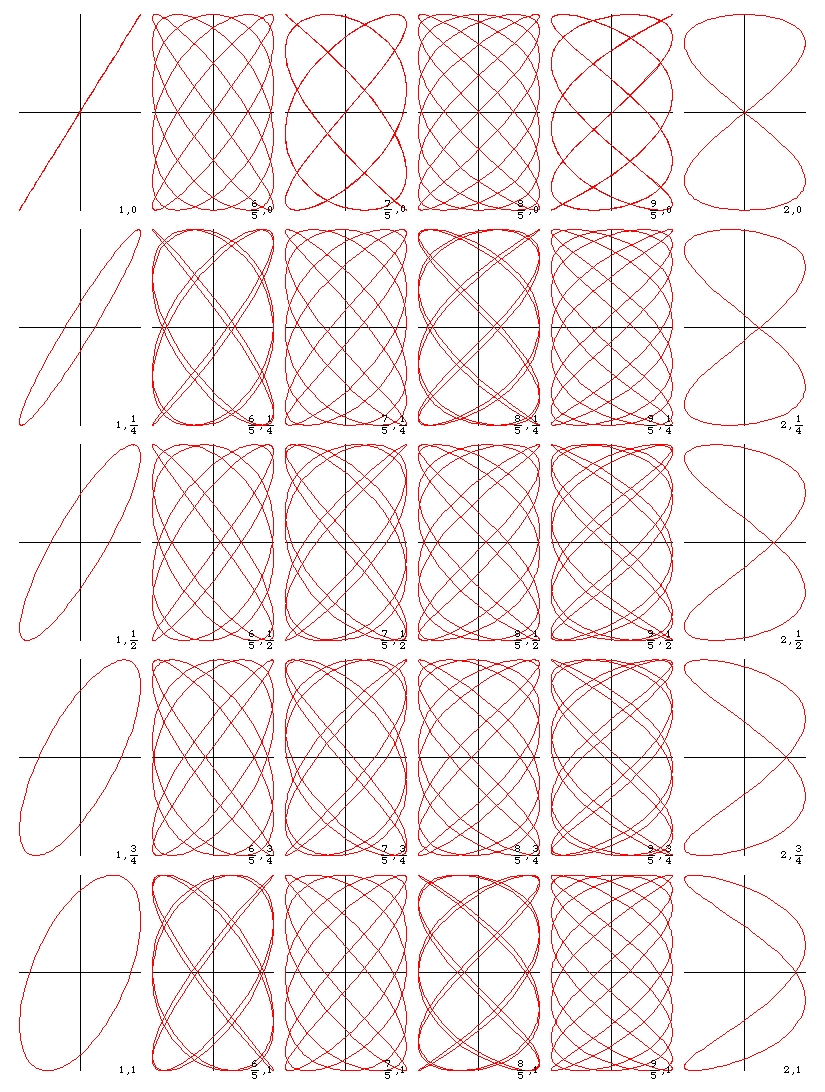# Lissajous (in the works)Varieties of Lissajous {a*Sin[b*t*2*π+c],Sin[t*2*π]}, where a is the inverse of golder ratio 1/ϕ ≈ 0.618, and b, c are indicated in the corner. lissajous.nb.

## Description

Lissajous is a family of curves, given by `{a*Sin[b*t+c], Sin[t]}` with 3 parameters.

In studying nature there often arises the wave motion `a*Sin[b*t+c]`. For example, the motion of a pendulum. Lissajous is two such motions in perpendicular directions:

```{a1*Sin[b1*t+c1],
a2*Sin[b2*t+c2]}```

Changing the parameter by replacing t→1/b1*t then t→c2, then scale by 1/a2, we can simplify the parameters down to `{a*Sin[b*t+c], Sin[t]}`.

## History

Studied by Nathaniel Bowditch in 1815 and Jules Antoine Lissajous (1822 to 1880).

## Formula

Parametric: `{a*Sin[b*t+c], Sin[t]}`

The parameter a stretches the curve in one direction. Parameters b and c are more interesting. The period of the curve is the least common multiple of the two components, that is, LCM[2*π/b,2*π].